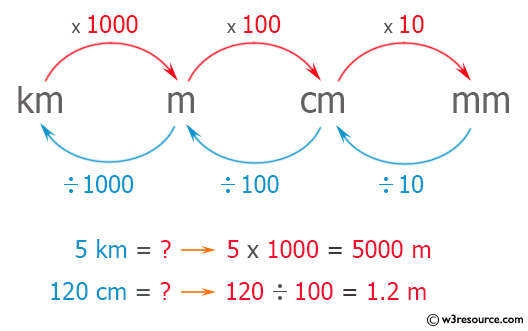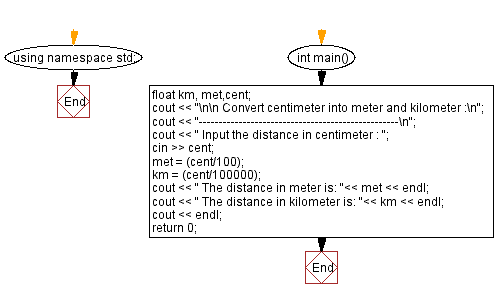﻿ C++ : Convert centimeter into meter and kilometer

# C++ Exercises: Enter length in centimeter and convert it into meter and kilometer

## C++ Basic: Exercise-50 with Solution

Write a C++ program to enter length in centimeters and convert it into meters and kilometers.

Pictorial Presentation:Sample Solution:

C++ Code :

``````#include<iostream>
using namespace std;

int main()
{
float km, met,cent;
cout << "\n\n Convert centimeter into meter and kilometer :\n";
cout << "--------------------------------------------------\n";
cout << " Input the distance in centimeter : ";
cin >> cent;
met = (cent/100);
km = (cent/100000);
cout << " The distance in meter is: "<< met << endl;
cout << " The distance in kilometer is: "<< km << endl;
cout << endl;
return 0;
}
``````

Sample Output:

``` Convert centimeter into meter and kilometer :
--------------------------------------------------
Input the distance in centimeter : 250000
The distance in meter is: 2500
The distance in kilometer is: 2.5
```

Flowchart:C++ Code Editor:

What is the difficulty level of this exercise?

﻿

## C++ Programming: Tips of the Day

Why is there no std::stou?

The most pat answer would be that the C library has no corresponding "strtou", and the C++11 string functions are all just thinly veiled wrappers around the C library functions: The std::sto* functions mirror strto*, and the std::to_string functions use sprintf.

Ref: https://bit.ly/3wtz2qA

We are closing our Disqus commenting system for some maintenanace issues. You may write to us at reach[at]yahoo[dot]com or visit us at Facebook